Скачать презентацию CHAPTER 15 Chemical Equilibrium Equilibrium Consider a

eda354160d7248bb8b284a577ce3e670.ppt

• Количество слайдов: 36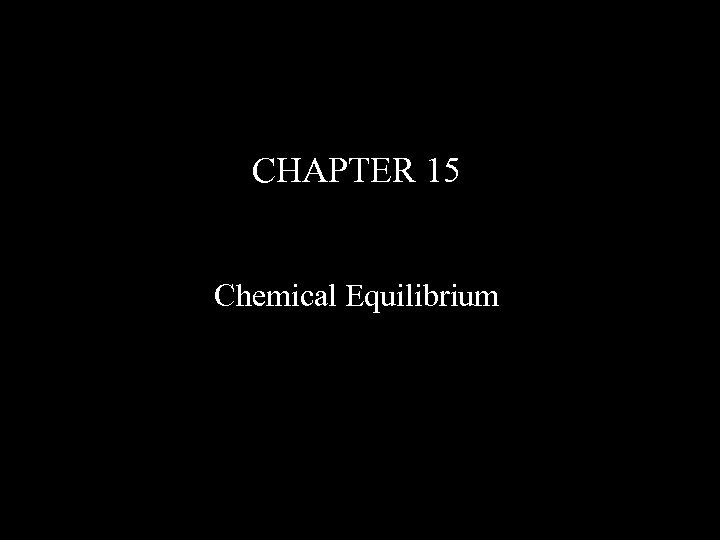CHAPTER 15 Chemical Equilibrium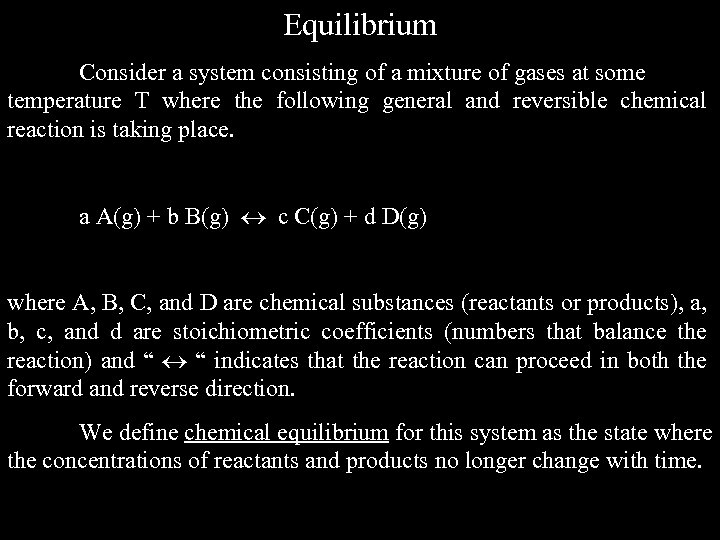Equilibrium Consider a system consisting of a mixture of gases at some temperature T where the following general and reversible chemical reaction is taking place. a A(g) + b B(g) c C(g) + d D(g) where A, B, C, and D are chemical substances (reactants or products), a, b, c, and d are stoichiometric coefficients (numbers that balance the reaction) and “ “ indicates that the reaction can proceed in both the forward and reverse direction. We define chemical equilibrium for this system as the state where the concentrations of reactants and products no longer change with time.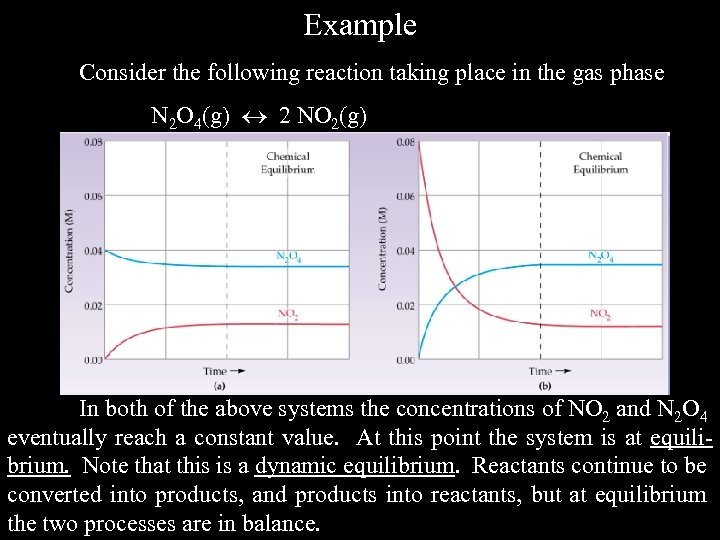Example Consider the following reaction taking place in the gas phase N 2 O 4(g) 2 NO 2(g) In both of the above systems the concentrations of NO 2 and N 2 O 4 eventually reach a constant value. At this point the system is at equilibrium. Note that this is a dynamic equilibrium. Reactants continue to be converted into products, and products into reactants, but at equilibrium the two processes are in balance.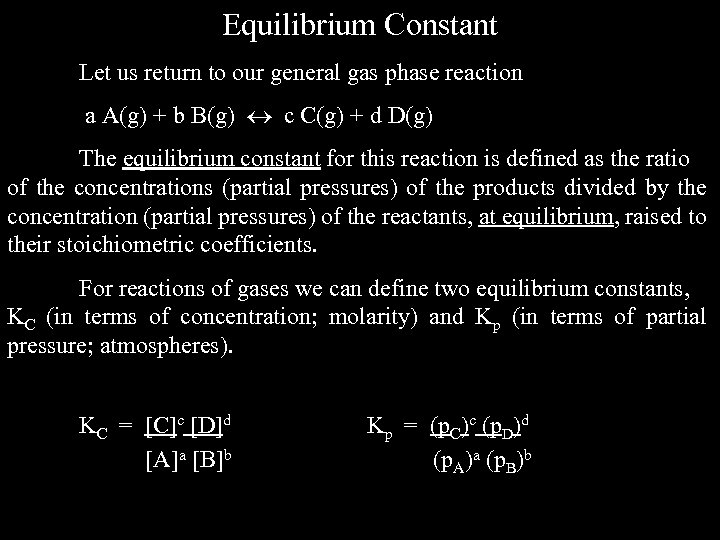Equilibrium Constant Let us return to our general gas phase reaction a A(g) + b B(g) c C(g) + d D(g) The equilibrium constant for this reaction is defined as the ratio of the concentrations (partial pressures) of the products divided by the concentration (partial pressures) of the reactants, at equilibrium, raised to their stoichiometric coefficients. For reactions of gases we can define two equilibrium constants, KC (in terms of concentration; molarity) and Kp (in terms of partial pressure; atmospheres). KC = [C]c [D]d [A]a [B]b Kp = (p. C)c (p. D)d (p. A)a (p. B)b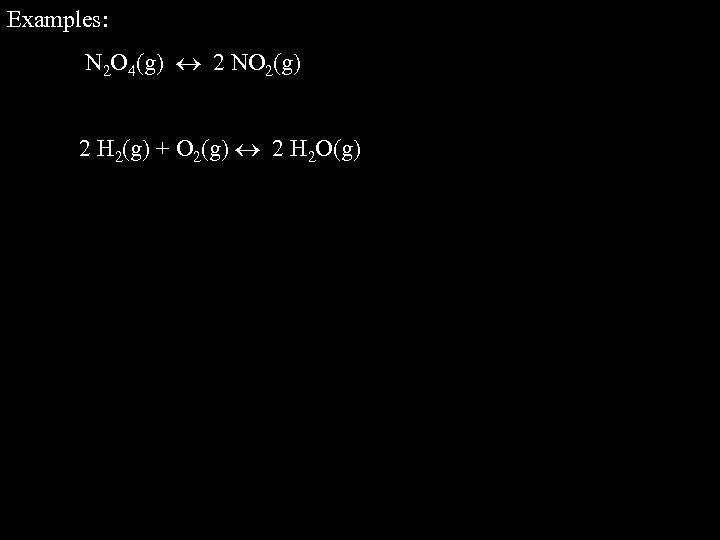Examples: N 2 O 4(g) 2 NO 2(g) 2 H 2(g) + O 2(g) 2 H 2 O(g)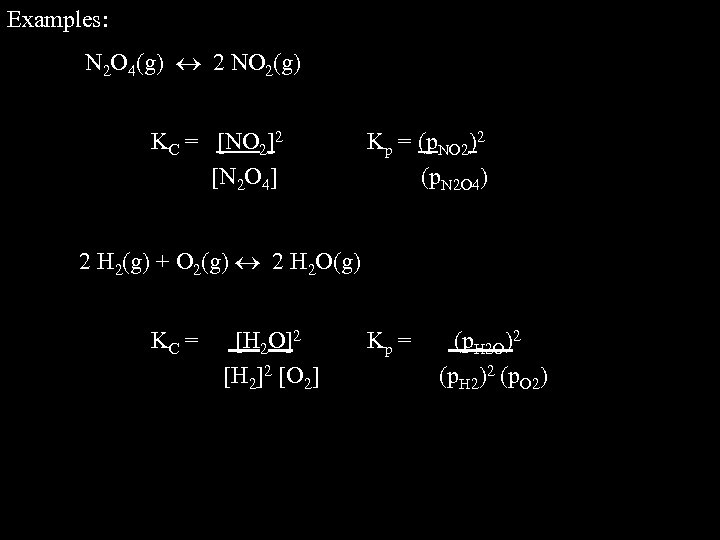Examples: N 2 O 4(g) 2 NO 2(g) KC = [NO 2]2 [N 2 O 4] Kp = (p. NO 2)2 (p. N 2 O 4) 2 H 2(g) + O 2(g) 2 H 2 O(g) KC = [H 2 O]2 [H 2]2 [O 2] Kp = (p. H 2 O)2 (p. H 2)2 (p. O 2)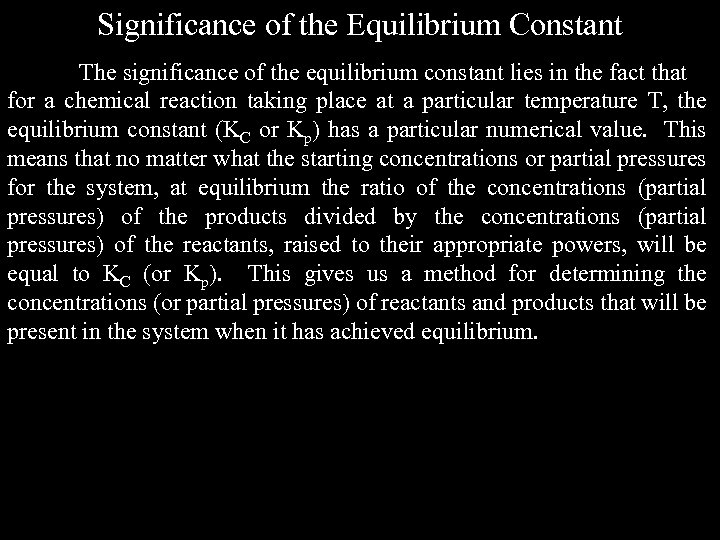Significance of the Equilibrium Constant The significance of the equilibrium constant lies in the fact that for a chemical reaction taking place at a particular temperature T, the equilibrium constant (KC or Kp) has a particular numerical value. This means that no matter what the starting concentrations or partial pressures for the system, at equilibrium the ratio of the concentrations (partial pressures) of the products divided by the concentrations (partial pressures) of the reactants, raised to their appropriate powers, will be equal to KC (or Kp). This gives us a method for determining the concentrations (or partial pressures) of reactants and products that will be present in the system when it has achieved equilibrium.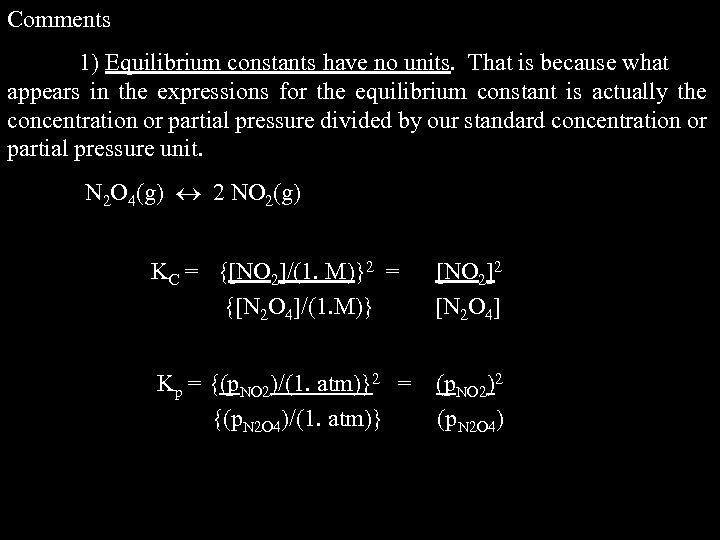Comments 1) Equilibrium constants have no units. That is because what appears in the expressions for the equilibrium constant is actually the concentration or partial pressure divided by our standard concentration or partial pressure unit. N 2 O 4(g) 2 NO 2(g) KC = {[NO 2]/(1. M)}2 = {[N 2 O 4]/(1. M)} [NO 2]2 [N 2 O 4] Kp = {(p. NO 2)/(1. atm)}2 = {(p. N 2 O 4)/(1. atm)} (p. NO 2)2 (p. N 2 O 4)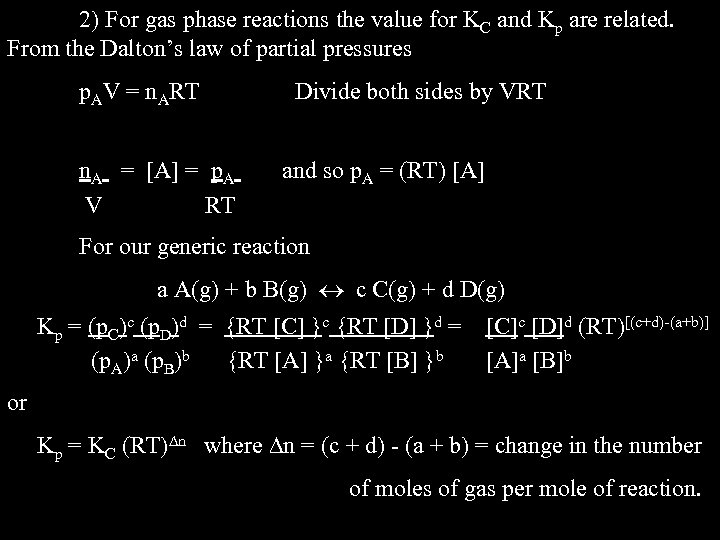2) For gas phase reactions the value for KC and Kp are related. From the Dalton’s law of partial pressures p. AV = n. ART n. A = [A] = p. A V RT Divide both sides by VRT and so p. A = (RT) [A] For our generic reaction a A(g) + b B(g) c C(g) + d D(g) Kp = (p. C)c (p. D)d = {RT [C] }c {RT [D] }d = (p. A)a (p. B)b {RT [A] }a {RT [B] }b [C]c [D]d (RT)[(c+d)-(a+b)] [A]a [B]b or Kp = KC (RT) n where n = (c + d) - (a + b) = change in the number of moles of gas per mole of reaction.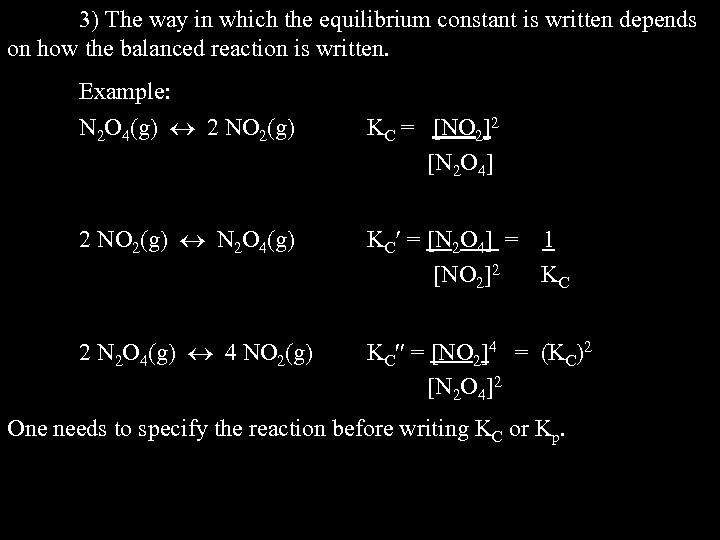3) The way in which the equilibrium constant is written depends on how the balanced reaction is written. Example: N 2 O 4(g) 2 NO 2(g) KC = [NO 2]2 [N 2 O 4] 2 NO 2(g) N 2 O 4(g) KC = [N 2 O 4] = 1 [NO 2]2 KC 2 N 2 O 4(g) 4 NO 2(g) KC = [NO 2]4 = (KC)2 [N 2 O 4]2 One needs to specify the reaction before writing KC or Kp.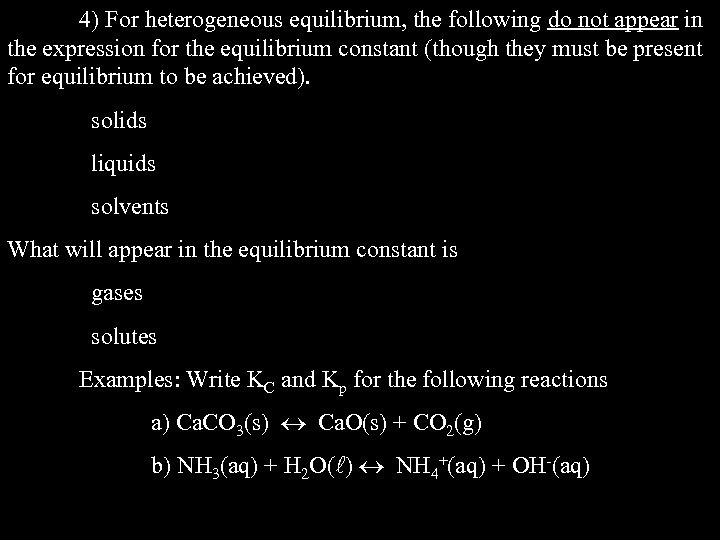4) For heterogeneous equilibrium, the following do not appear in the expression for the equilibrium constant (though they must be present for equilibrium to be achieved). solids liquids solvents What will appear in the equilibrium constant is gases solutes Examples: Write KC and Kp for the following reactions a) Ca. CO 3(s) Ca. O(s) + CO 2(g) b) NH 3(aq) + H 2 O( ) NH 4+(aq) + OH-(aq)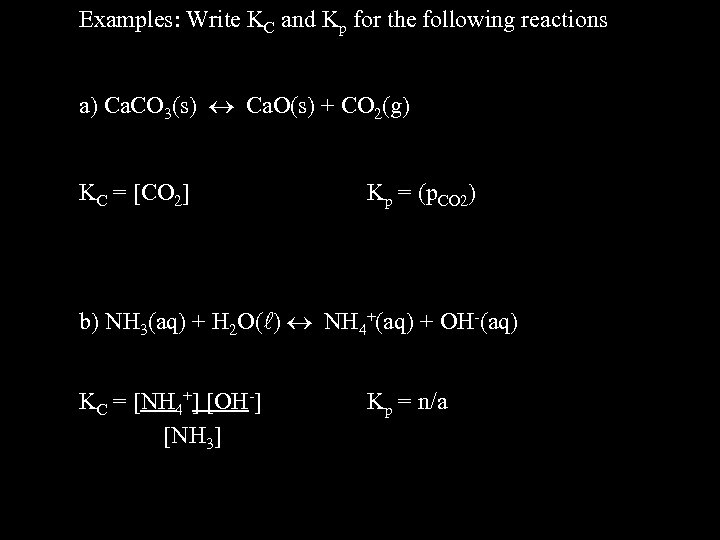Examples: Write KC and Kp for the following reactions a) Ca. CO 3(s) Ca. O(s) + CO 2(g) KC = [CO 2] Kp = (p. CO 2) b) NH 3(aq) + H 2 O( ) NH 4+(aq) + OH-(aq) KC = [NH 4+] [OH-] [NH 3] Kp = n/a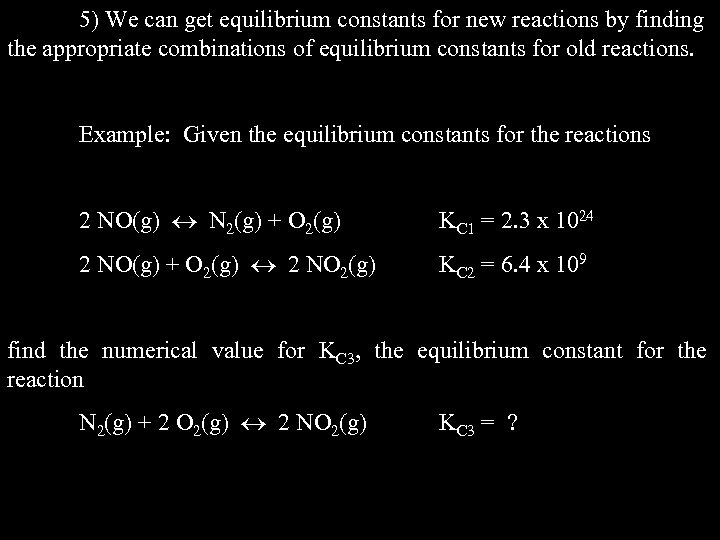5) We can get equilibrium constants for new reactions by finding the appropriate combinations of equilibrium constants for old reactions. Example: Given the equilibrium constants for the reactions 2 NO(g) N 2(g) + O 2(g) KC 1 = 2. 3 x 1024 2 NO(g) + O 2(g) 2 NO 2(g) KC 2 = 6. 4 x 109 find the numerical value for KC 3, the equilibrium constant for the reaction N 2(g) + 2 O 2(g) 2 NO 2(g) KC 3 = ?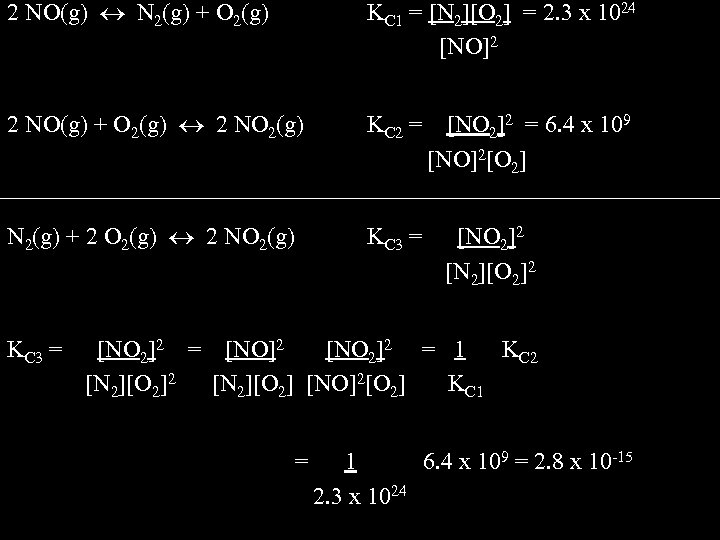2 NO(g) N 2(g) + O 2(g) KC 1 = [N 2][O 2] = 2. 3 x 1024 [NO]2 2 NO(g) + O 2(g) 2 NO 2(g) KC 2 = N 2(g) + 2 O 2(g) 2 NO 2(g) KC 3 = [NO 2]2 = 6. 4 x 109 [NO]2[O 2] [NO 2]2 [N 2][O 2]2 [NO 2]2 = [NO]2 [NO 2]2 = 1 KC 2 [N 2][O 2] [NO]2[O 2] KC 1 = 1 6. 4 x 109 = 2. 8 x 10 -15 2. 3 x 1024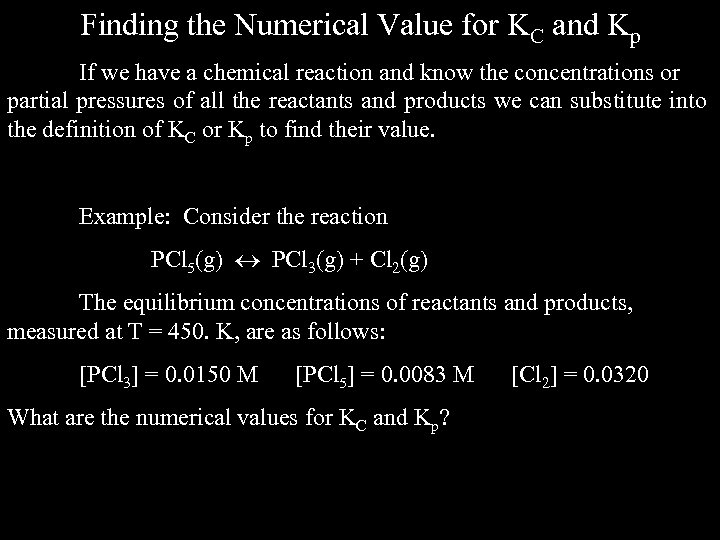Finding the Numerical Value for KC and Kp If we have a chemical reaction and know the concentrations or partial pressures of all the reactants and products we can substitute into the definition of KC or Kp to find their value. Example: Consider the reaction PCl 5(g) PCl 3(g) + Cl 2(g) The equilibrium concentrations of reactants and products, measured at T = 450. K, are as follows: [PCl 3] = 0. 0150 M [PCl 5] = 0. 0083 M What are the numerical values for KC and Kp? [Cl 2] = 0. 0320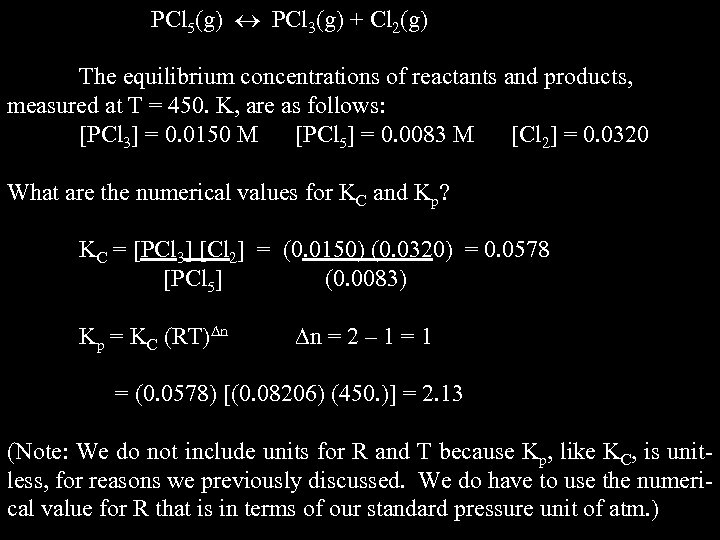PCl 5(g) PCl 3(g) + Cl 2(g) The equilibrium concentrations of reactants and products, measured at T = 450. K, are as follows: [PCl 3] = 0. 0150 M [PCl 5] = 0. 0083 M [Cl 2] = 0. 0320 What are the numerical values for KC and Kp? KC = [PCl 3] [Cl 2] = (0. 0150) (0. 0320) = 0. 0578 [PCl 5] (0. 0083) Kp = KC (RT) n n = 2 – 1 = (0. 0578) [(0. 08206) (450. )] = 2. 13 (Note: We do not include units for R and T because Kp, like KC, is unitless, for reasons we previously discussed. We do have to use the numerical value for R that is in terms of our standard pressure unit of atm. )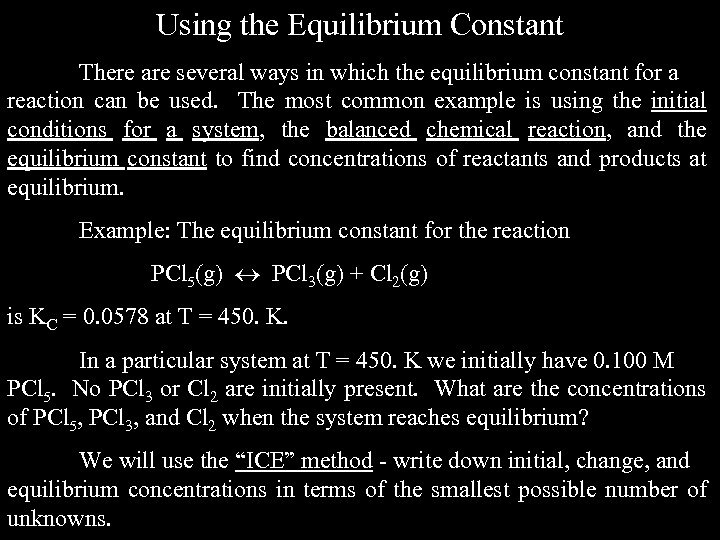Using the Equilibrium Constant There are several ways in which the equilibrium constant for a reaction can be used. The most common example is using the initial conditions for a system, the balanced chemical reaction, and the equilibrium constant to find concentrations of reactants and products at equilibrium. Example: The equilibrium constant for the reaction PCl 5(g) PCl 3(g) + Cl 2(g) is KC = 0. 0578 at T = 450. K. In a particular system at T = 450. K we initially have 0. 100 M PCl 5. No PCl 3 or Cl 2 are initially present. What are the concentrations of PCl 5, PCl 3, and Cl 2 when the system reaches equilibrium? We will use the “ICE” method - write down initial, change, and equilibrium concentrations in terms of the smallest possible number of unknowns.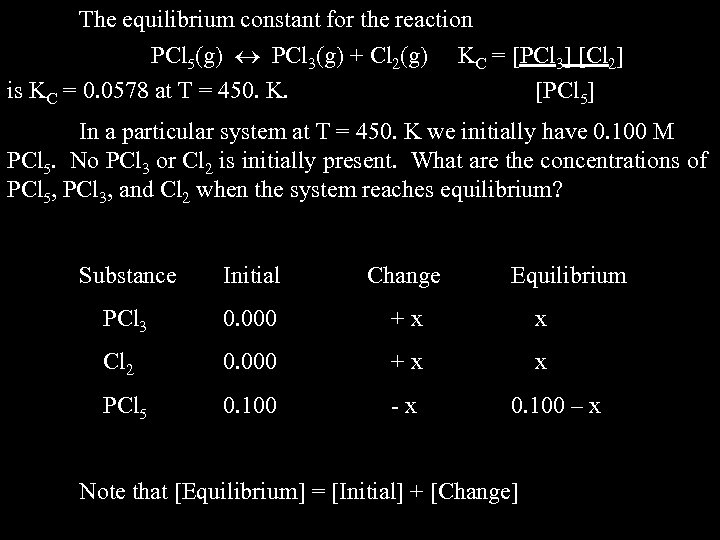The equilibrium constant for the reaction PCl 5(g) PCl 3(g) + Cl 2(g) is KC = 0. 0578 at T = 450. K. KC = [PCl 3] [Cl 2] [PCl 5] In a particular system at T = 450. K we initially have 0. 100 M PCl 5. No PCl 3 or Cl 2 is initially present. What are the concentrations of PCl 5, PCl 3, and Cl 2 when the system reaches equilibrium? Substance Initial Change Equilibrium PCl 3 0. 000 +x x Cl 2 0. 000 +x x PCl 5 0. 100 -x 0. 100 – x Note that [Equilibrium] = [Initial] + [Change]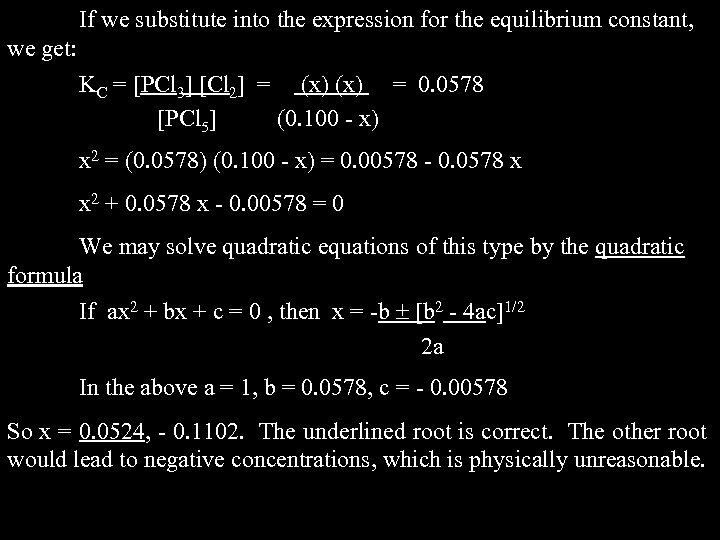If we substitute into the expression for the equilibrium constant, we get: KC = [PCl 3] [Cl 2] = (x) = 0. 0578 [PCl 5] (0. 100 - x) x 2 = (0. 0578) (0. 100 - x) = 0. 00578 - 0. 0578 x x 2 + 0. 0578 x - 0. 00578 = 0 We may solve quadratic equations of this type by the quadratic formula If ax 2 + bx + c = 0 , then x = -b [b 2 - 4 ac]1/2 2 a In the above a = 1, b = 0. 0578, c = - 0. 00578 So x = 0. 0524, - 0. 1102. The underlined root is correct. The other root would lead to negative concentrations, which is physically unreasonable.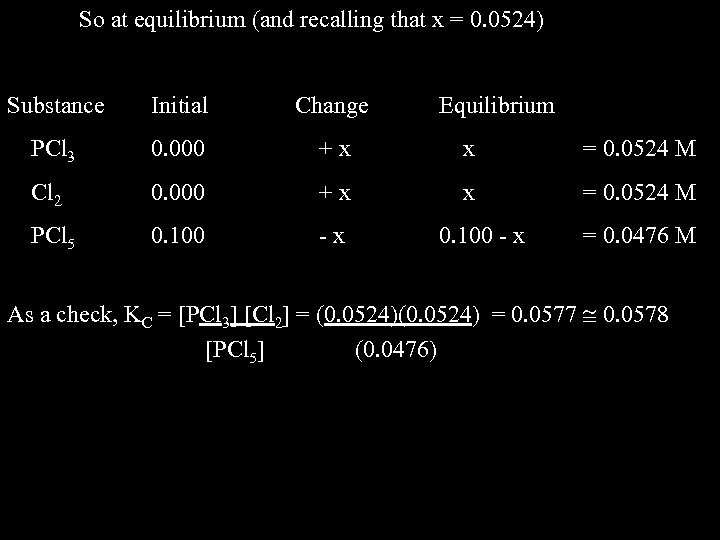So at equilibrium (and recalling that x = 0. 0524) Substance Initial Change Equilibrium PCl 3 0. 000 +x x = 0. 0524 M Cl 2 0. 000 +x x = 0. 0524 M PCl 5 0. 100 -x 0. 100 - x = 0. 0476 M As a check, KC = [PCl 3] [Cl 2] = (0. 0524) = 0. 0577 0. 0578 [PCl 5] (0. 0476)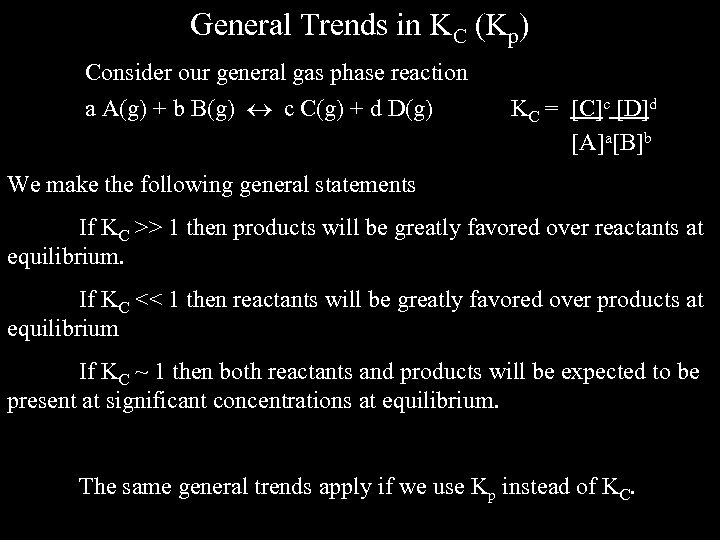General Trends in KC (Kp) Consider our general gas phase reaction a A(g) + b B(g) c C(g) + d D(g) KC = [C]c [D]d [A]a[B]b We make the following general statements If KC >> 1 then products will be greatly favored over reactants at equilibrium. If KC << 1 then reactants will be greatly favored over products at equilibrium If KC ~ 1 then both reactants and products will be expected to be present at significant concentrations at equilibrium. The same general trends apply if we use Kp instead of KC.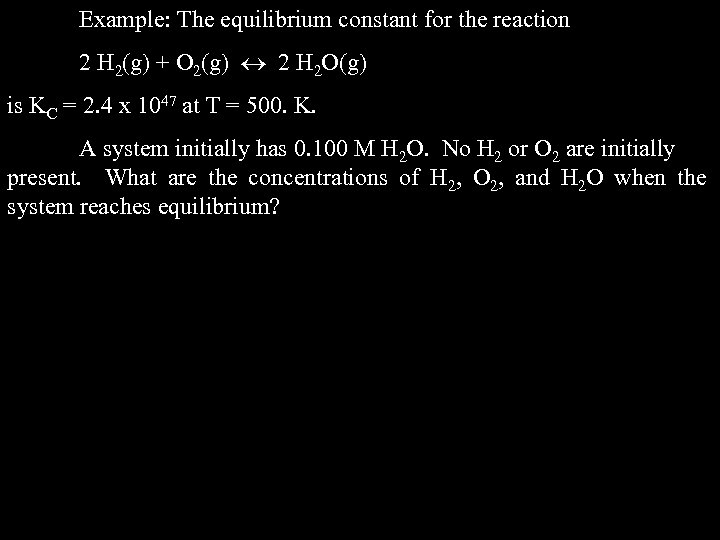Example: The equilibrium constant for the reaction 2 H 2(g) + O 2(g) 2 H 2 O(g) is KC = 2. 4 x 1047 at T = 500. K. A system initially has 0. 100 M H 2 O. No H 2 or O 2 are initially present. What are the concentrations of H 2, O 2, and H 2 O when the system reaches equilibrium?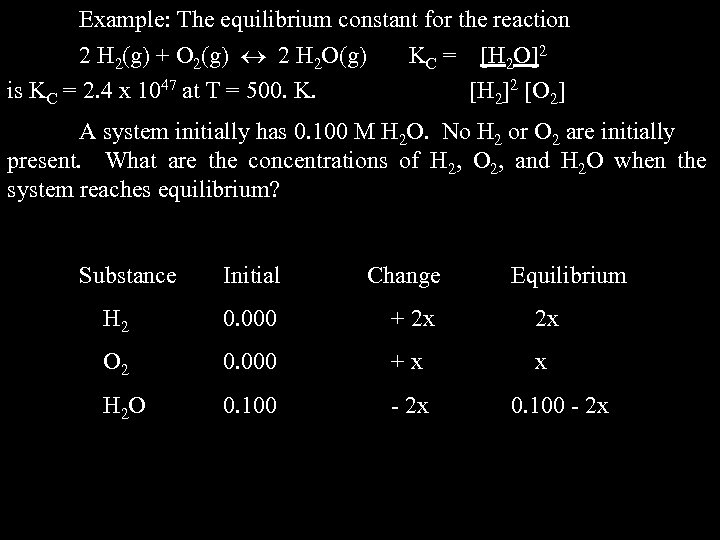Example: The equilibrium constant for the reaction 2 H 2(g) + O 2(g) 2 H 2 O(g) is KC = 2. 4 x 1047 at T = 500. K. KC = [H 2 O]2 [H 2]2 [O 2] A system initially has 0. 100 M H 2 O. No H 2 or O 2 are initially present. What are the concentrations of H 2, O 2, and H 2 O when the system reaches equilibrium? Substance Initial Change Equilibrium H 2 0. 000 + 2 x 2 x O 2 0. 000 +x x H 2 O 0. 100 - 2 x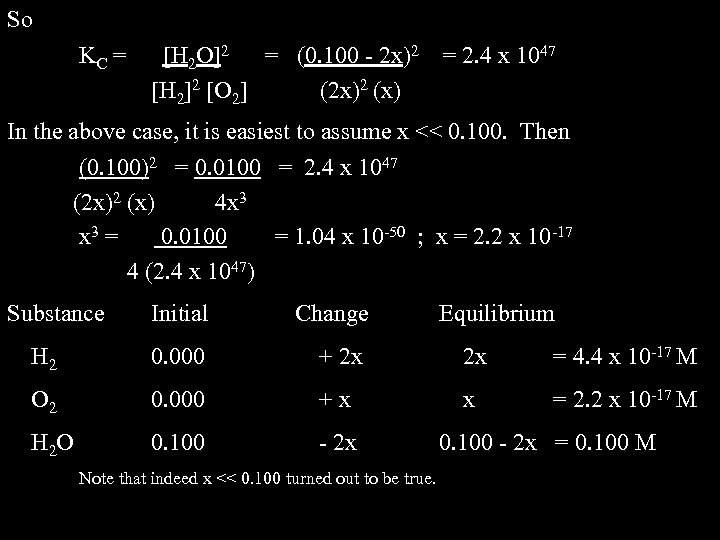So KC = [H 2 O]2 = (0. 100 - 2 x)2 [H 2]2 [O 2] (2 x)2 (x) = 2. 4 x 1047 In the above case, it is easiest to assume x << 0. 100. Then (0. 100)2 = 0. 0100 = 2. 4 x 1047 (2 x)2 (x) 4 x 3 = 0. 0100 = 1. 04 x 10 -50 ; x = 2. 2 x 10 -17 4 (2. 4 x 1047) Substance Initial Change H 2 0. 000 + 2 x 2 x = 4. 4 x 10 -17 M O 2 0. 000 +x x = 2. 2 x 10 -17 M H 2 O 0. 100 - 2 x Note that indeed x << 0. 100 turned out to be true. Equilibrium 0. 100 - 2 x = 0. 100 M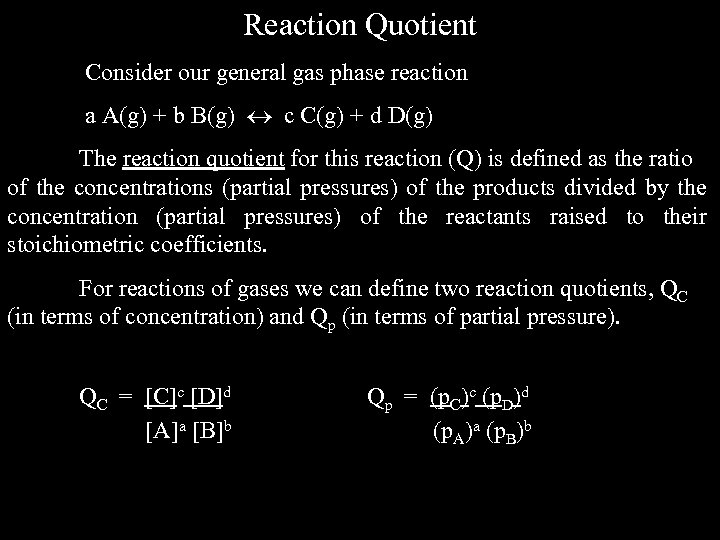Reaction Quotient Consider our general gas phase reaction a A(g) + b B(g) c C(g) + d D(g) The reaction quotient for this reaction (Q) is defined as the ratio of the concentrations (partial pressures) of the products divided by the concentration (partial pressures) of the reactants raised to their stoichiometric coefficients. For reactions of gases we can define two reaction quotients, QC (in terms of concentration) and Qp (in terms of partial pressure). QC = [C]c [D]d [A]a [B]b Qp = (p. C)c (p. D)d (p. A)a (p. B)b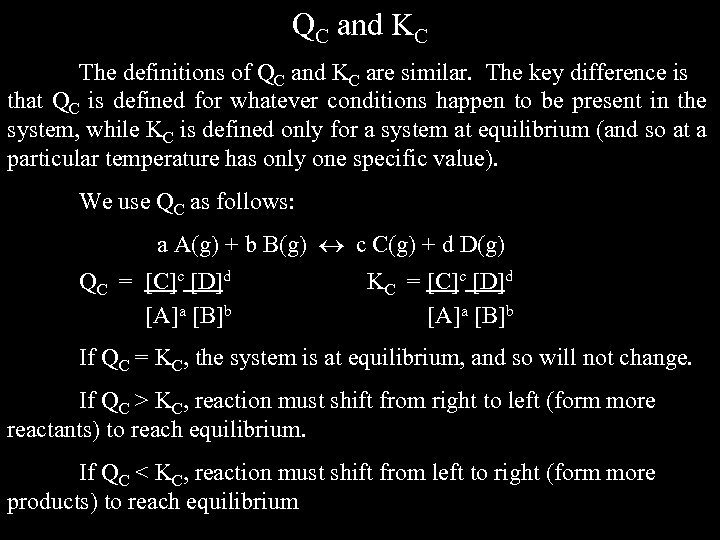QC and KC The definitions of QC and KC are similar. The key difference is that QC is defined for whatever conditions happen to be present in the system, while KC is defined only for a system at equilibrium (and so at a particular temperature has only one specific value). We use QC as follows: a A(g) + b B(g) c C(g) + d D(g) QC = [C]c [D]d [A]a [B]b KC = [C]c [D]d [A]a [B]b If QC = KC, the system is at equilibrium, and so will not change. If QC > KC, reaction must shift from right to left (form more reactants) to reach equilibrium. If QC < KC, reaction must shift from left to right (form more products) to reach equilibrium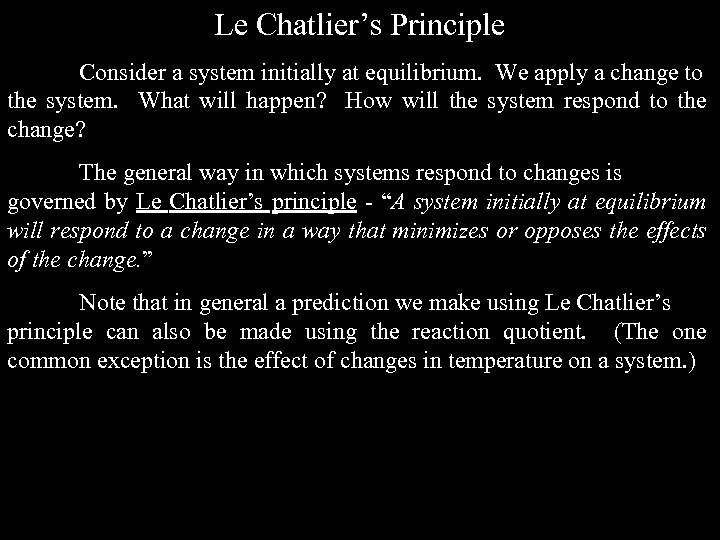Le Chatlier’s Principle Consider a system initially at equilibrium. We apply a change to the system. What will happen? How will the system respond to the change? The general way in which systems respond to changes is governed by Le Chatlier’s principle - “A system initially at equilibrium will respond to a change in a way that minimizes or opposes the effects of the change. ” Note that in general a prediction we make using Le Chatlier’s principle can also be made using the reaction quotient. (The one common exception is the effect of changes in temperature on a system. )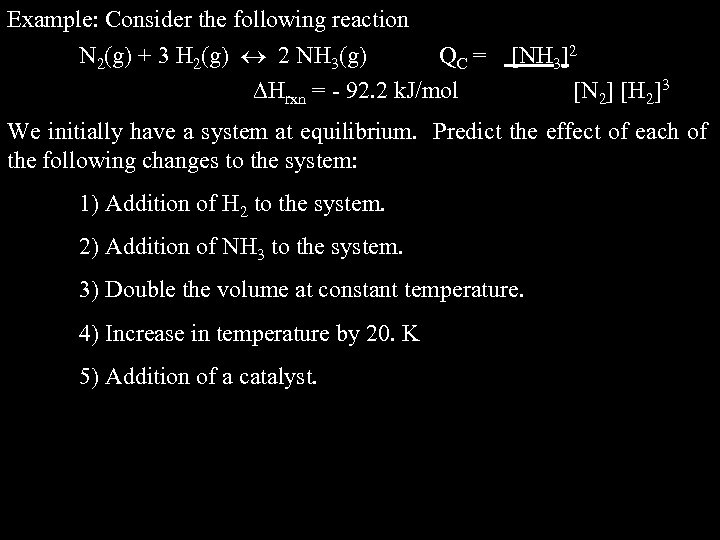Example: Consider the following reaction N 2(g) + 3 H 2(g) 2 NH 3(g) QC = Hrxn = - 92. 2 k. J/mol [NH 3]2 [N 2] [H 2]3 We initially have a system at equilibrium. Predict the effect of each of the following changes to the system: 1) Addition of H 2 to the system. 2) Addition of NH 3 to the system. 3) Double the volume at constant temperature. 4) Increase in temperature by 20. K 5) Addition of a catalyst.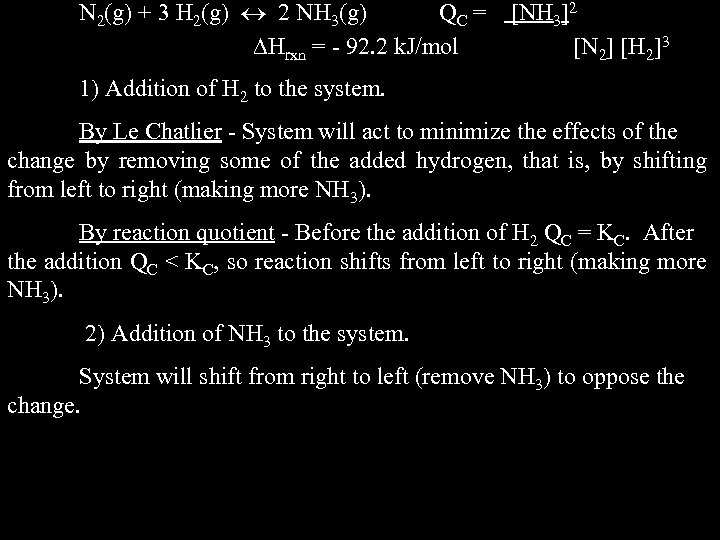N 2(g) + 3 H 2(g) 2 NH 3(g) QC = Hrxn = - 92. 2 k. J/mol [NH 3]2 [N 2] [H 2]3 1) Addition of H 2 to the system. By Le Chatlier - System will act to minimize the effects of the change by removing some of the added hydrogen, that is, by shifting from left to right (making more NH 3). By reaction quotient - Before the addition of H 2 QC = KC. After the addition QC < KC, so reaction shifts from left to right (making more NH 3). 2) Addition of NH 3 to the system. System will shift from right to left (remove NH 3) to oppose the change.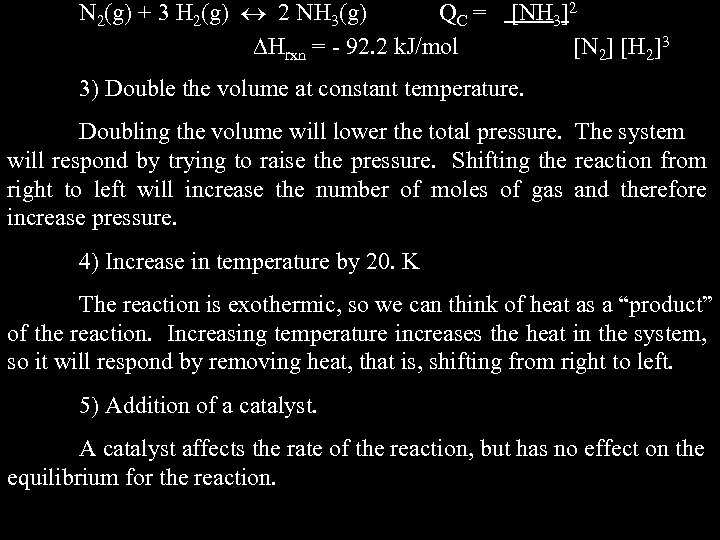N 2(g) + 3 H 2(g) 2 NH 3(g) QC = Hrxn = - 92. 2 k. J/mol [NH 3]2 [N 2] [H 2]3 3) Double the volume at constant temperature. Doubling the volume will lower the total pressure. The system will respond by trying to raise the pressure. Shifting the reaction from right to left will increase the number of moles of gas and therefore increase pressure. 4) Increase in temperature by 20. K The reaction is exothermic, so we can think of heat as a “product” of the reaction. Increasing temperature increases the heat in the system, so it will respond by removing heat, that is, shifting from right to left. 5) Addition of a catalyst. A catalyst affects the rate of the reaction, but has no effect on the equilibrium for the reaction.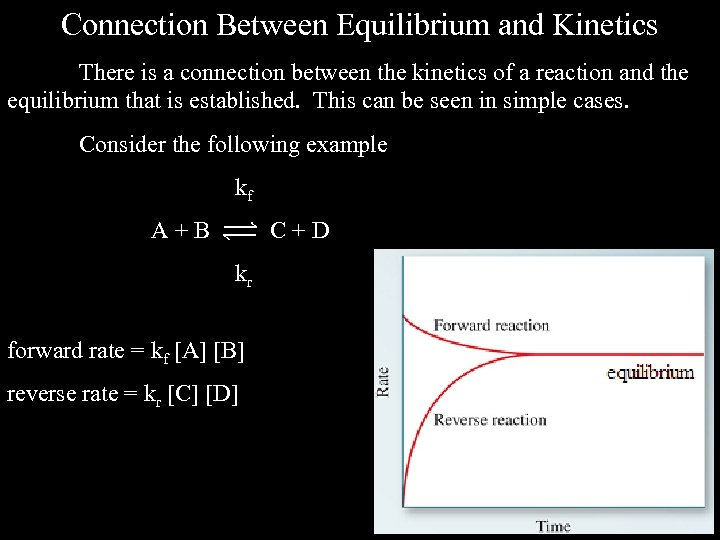Connection Between Equilibrium and Kinetics There is a connection between the kinetics of a reaction and the equilibrium that is established. This can be seen in simple cases. Consider the following example kf A+B C+D kr forward rate = kf [A] [B] reverse rate = kr [C] [D]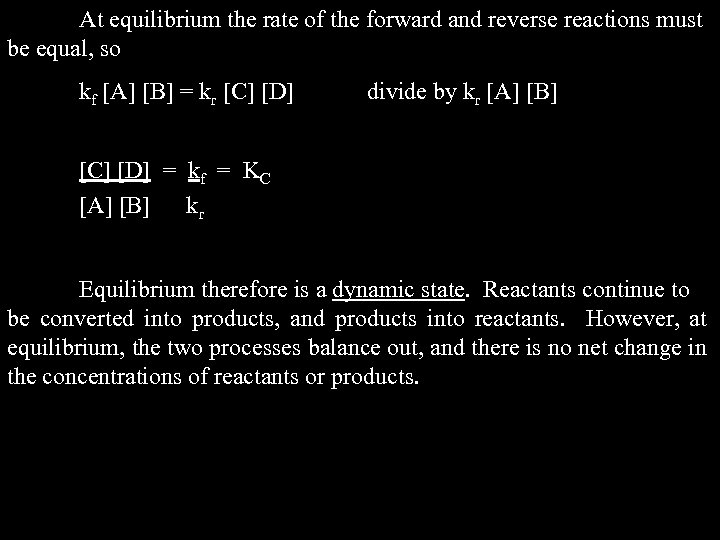At equilibrium the rate of the forward and reverse reactions must be equal, so kf [A] [B] = kr [C] [D] divide by kr [A] [B] [C] [D] = kf = KC [A] [B] kr Equilibrium therefore is a dynamic state. Reactants continue to be converted into products, and products into reactants. However, at equilibrium, the two processes balance out, and there is no net change in the concentrations of reactants or products.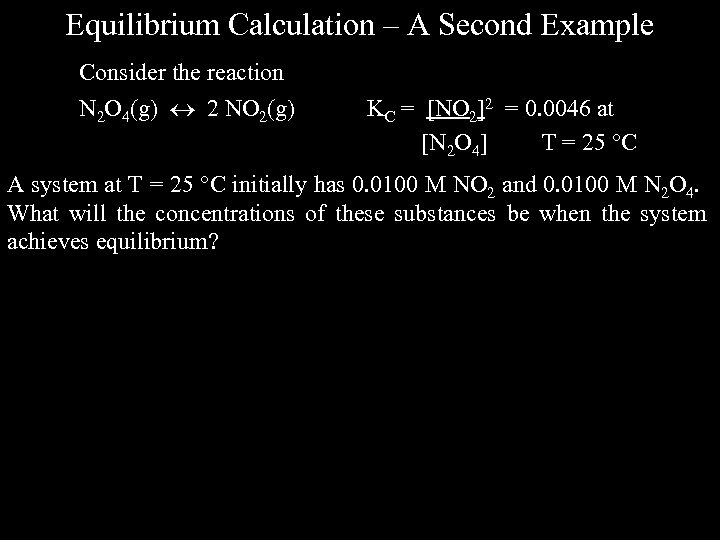Equilibrium Calculation – A Second Example Consider the reaction N 2 O 4(g) 2 NO 2(g) KC = [NO 2]2 = 0. 0046 at [N 2 O 4] T = 25 C A system at T = 25 C initially has 0. 0100 M NO 2 and 0. 0100 M N 2 O 4. What will the concentrations of these substances be when the system achieves equilibrium?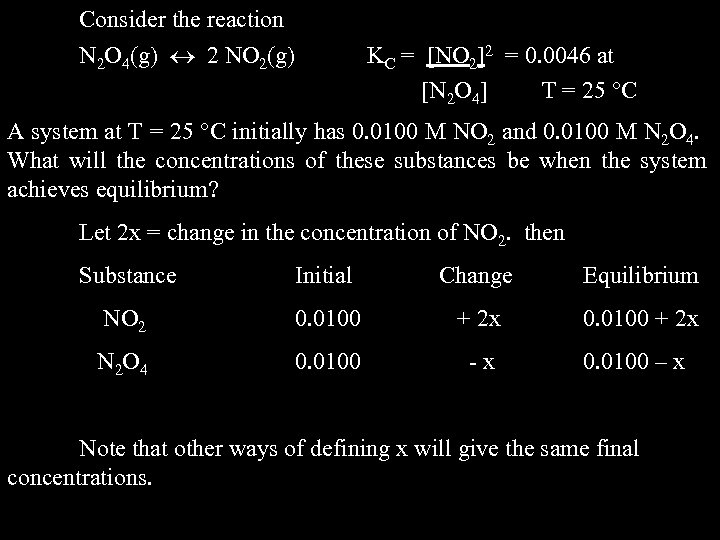Consider the reaction N 2 O 4(g) 2 NO 2(g) KC = [NO 2]2 = 0. 0046 at [N 2 O 4] T = 25 C A system at T = 25 C initially has 0. 0100 M NO 2 and 0. 0100 M N 2 O 4. What will the concentrations of these substances be when the system achieves equilibrium? Let 2 x = change in the concentration of NO 2. then Substance Initial Change Equilibrium NO 2 0. 0100 + 2 x N 2 O 4 0. 0100 -x 0. 0100 – x Note that other ways of defining x will give the same final concentrations.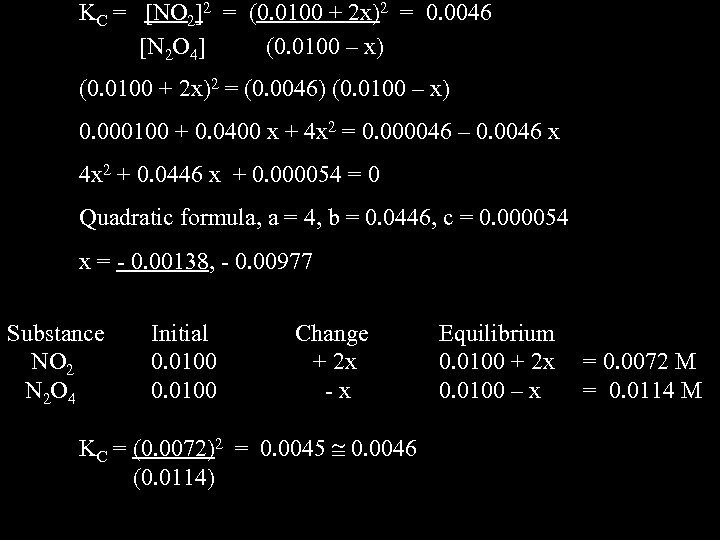KC = [NO 2]2 = (0. 0100 + 2 x)2 = 0. 0046 [N 2 O 4] (0. 0100 – x) (0. 0100 + 2 x)2 = (0. 0046) (0. 0100 – x) 0. 000100 + 0. 0400 x + 4 x 2 = 0. 000046 – 0. 0046 x 4 x 2 + 0. 0446 x + 0. 000054 = 0 Quadratic formula, a = 4, b = 0. 0446, c = 0. 000054 x = - 0. 00138, - 0. 00977 Substance NO 2 N 2 O 4 Initial 0. 0100 Change + 2 x -x KC = (0. 0072)2 = 0. 0045 0. 0046 (0. 0114) Equilibrium 0. 0100 + 2 x 0. 0100 – x = 0. 0072 M = 0. 0114 M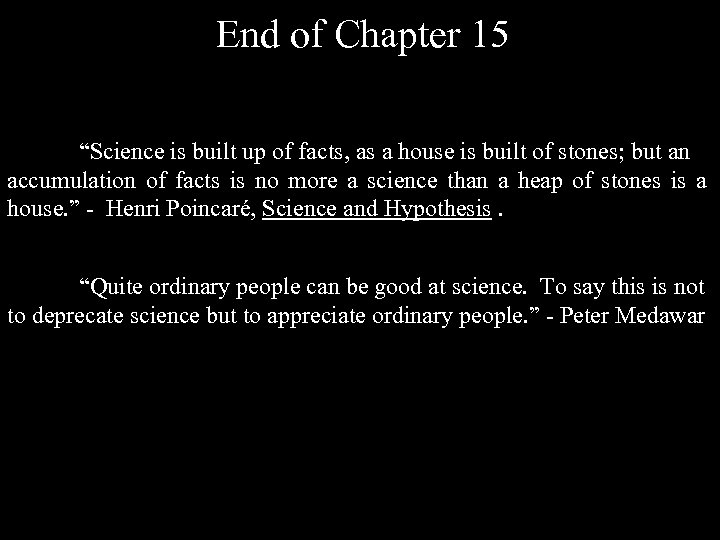End of Chapter 15 “Science is built up of facts, as a house is built of stones; but an accumulation of facts is no more a science than a heap of stones is a house. ” - Henri Poincaré, Science and Hypothesis. “Quite ordinary people can be good at science. To say this is not to deprecate science but to appreciate ordinary people. ” - Peter Medawar